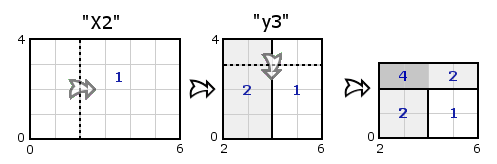### Problem Statement

You have been given a width-by-height rectangular piece of paper to fold. For the sake of clarity, assume the paper is on a grid such that the width is parallel to the x-axis and the height is parallel to the y-axis. In addition, assume the paper lies entirely in non-negative coordinates, with one corner on the point (0,0). In order, you will perform a sequence of folds on the paper. More precisely, after concatenating the elements of folds you will have a single space-delimited list of single folds. Each single fold will be formatted as (quotes for clarity) "TypeCoordinate", where Type is either 'X', 'x', 'Y', or 'y', and Coordinate is an integer. Uppercase types denote folds in the positive direction. For example, "X10" denotes a fold along a vertical axis with x-coordinate 10 in the positive direction. Positive direction means that the portion of the paper to the left of the crease is folded toward the right (toward the positive x direction). Positive direction would denote folding upward had the crease been along a horizontal axis. All folds are considered ideal in that they fold all layers of the paper and leave it perfectly flat. If a crease is given that doesn't intersect the interior of the paper (edges do not count), no fold occurs. Once all folds are complete, return the thickness of the thickest part of the paper. The thickness at a particular point is the number of layers of paper at that point.

### Definition

 Class: PaperThickness Method: maxThickness Parameters: String[], int, int Returns: long Method signature: long maxThickness(String[] folds, int width, int height) (be sure your method is public)

### Constraints

-width will be between 1 and 100000, inclusive.
-height will be between 1 and 100000, inclusive.
-folds will contain between 1 and 50 elements, inclusive.
-Each element of folds will contain between 1 and 50 characters, inclusive.
-Once concatenated, the elements of folds will form a single-space delimited list of folds with no leading or trailing spaces. Each fold will be formatted as (quotes for clarity) "TypeCoordinate", where

1) Type is either 'X', 'x', 'Y' or 'y', and

2) Coordinate is an integer between -100000 and 100000, inclusive, with no extra leading zeros and a single leading '-' on negative values.

### Examples

0)

 `{"X1"}` `3` `3`
`Returns: 2`
 We begin with a square piece of paper with corners at (0,0) and (3,3). Here only a single fold occurs along the vertical line x=1.
1)

 `{"X0 x-1 Y-10"}` `3` `3`
`Returns: 1`
 The same piece of paper, but here the folds miss the interior.
2)

 `{"X2", " y3"}` `6` `4`
`Returns: 4`3)

 `{"X1 ", "x3"}` `5` `4`
`Returns: 3`
4)

 `{"x1 y1"}` `2` `2`
`Returns: 4`
 Here the 2x2 square is folded into a thicker 1x1 square.

#### Problem url:

http://www.topcoder.com/stat?c=problem_statement&pm=2942

#### Problem stats url:

http://www.topcoder.com/tc?module=ProblemDetail&rd=10842&pm=2942